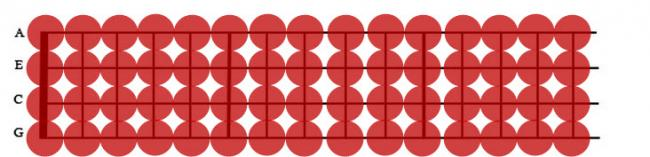#### Howdy, Stranger!

We are about to switch to a new forum software. Until then we have removed the registration on this forum.

# Create an ellipse depending on mouse coordinates

edited July 2014

I'm looking to create an ellipse at a certain coordinate depending on the Mouse coordinates. Depending on the mouse position, the dot will be placed on one of the positions of the fret board. Thanks for the help!

``````PImage img;
float X_Space = 32;
float Y_Space = 33;
float X_Base = 63;
float Y_Base = 28;

void setup(){
size(576,144);

}

void draw(){
image(img, 0, 0);
ellipse(X_Base+(4*X_Space),Y_Base,20,20);
``````Tagged:

• edited July 2014 Answer ✓

Just imagine a circle over each intersection and then use OverCircle() to determine the position. On mouse release loop over the positions to find the location. A release between circles will be a dead zone.

x,y is the line intersection, the diameter, the distance between frets``````       boolean overCircle(int x, int y,int diameter)
{
return (dist(x,y,mouseX,mouseY) < diameter/2);
}
``````
• edited July 2014 Answer ✓

Faster alternative for overCircle(): ;;)

``````// forum.processing.org/two/discussion/6247/
// do-these-functions-exist

// forum.processing.org/two/discussion/6250/
// create-an-ellipse-depending-on-mouse-coordinates

boolean isMouseWithinDiam(int x, int y, int d) {
return sq(x-mouseX) + sq(y-mouseY) <= sq(d>>1);
}
``````

Make it work, make it right, make it run faster...

Surprised no one suggested an optimization for calculating the column based on x, then the row, based on y, and checking the specific circle, and not looping over the 60 positions.

However for user interaction over a small number of fret positions it could be over-kill.

• no need for any distance calculations or any iterating - it's a grid with equal spacing in both directions (see lines 2-5).

just take the offset off the mouse position, integer divide it by the spacing. multiply it by the spacing and add the offset back on and that should've aligned it to one of the frets. (give or take half a spacing)

(think that's right - will supply a code example when i'm not at work)

• I've got an online example which places rects by offset in a double loop to form a grid: =:)

• which is completely unrelated. he HAS the grid, it's a png, it's attached in the question.

• ok, something like this. unfortunately the image attached doesn't quite match the numbers given - it might've been resized by the forum or during the download.

``````// ukulele
// acd 2014
PImage img;
float xSpace = 32;
float ySpace = 33;
float xBase = 45;
float yBase = 28;

void setup(){
size(576, 144);
smooth();
}

void draw(){
image(img, 0, 0);
// don't draw if off grid
if (mouseX > (xBase - RAD) && mouseY > (yBase - RAD)) {
// maths!
float ex = Math.round((mouseX - xBase) / xSpace) * xSpace + xBase;
float ey = Math.round((mouseY - yBase) / ySpace) * ySpace + yBase;